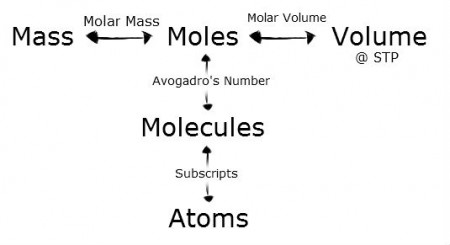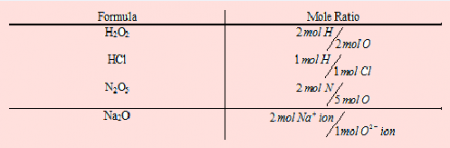Chemistry

# What is Mole?

A mole is defined as the amount of substance which contains different number of particles like; atoms, molecules, ions etc., as there are atoms in exactly 12.000 gm of Carbon-12.

One mole of Carbon-12 atom has a mass of exactly 12.000 grams and contains (6.02 * 10^23) atoms.

A mole is just a number like a Dozen. A dozen equals to 12 eggs, a gross of pencil equals to 144 pencil, similarly mole is equal to (6.02 * 10^23) atoms, here is Avogadro Constant. Mole is also known as chemist dozen.

The value of (6.02 * 10^23) is known as Avogadro Constant, after the Italian scientist who first recognized the importance of the mass/number relationship.Main concepts:

• 6.02 x 1023 of anything.
• The formula mass in grams of a substance contains one mole of particles.
• Na = Avogadro’s Number = 6.02 x 1023 Spreading a “mole” of marbles over the entire surface of the earth would produce a layer about three miles thick.